## 2D Transformations in Computer Graphics-

We have discussed-

• Transformation is a process of modifying and re-positioning the existing graphics.
• 2D Transformations take place in a two dimensional plane.

In computer graphics, various transformation techniques are-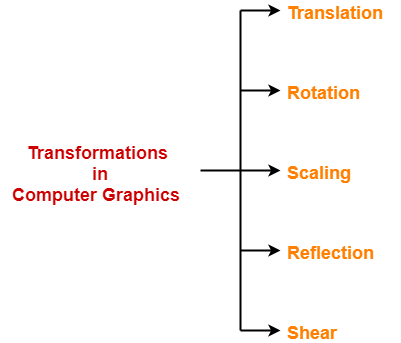## 2D Shearing in Computer Graphics-

 In Computer graphics,2D Shearing is an ideal technique to change the shape of an existing object in a two dimensional plane.

In a two dimensional plane, the object size can be changed along X direction as well as Y direction.

So, there are two versions of shearing-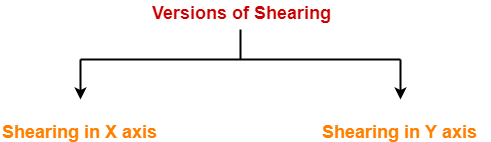1. Shearing in X direction
2. Shearing in Y direction

Consider a point object O has to be sheared in a 2D plane.

Let-

• Initial coordinates of the object O = (Xold, Yold)
• Shearing parameter towards X direction = Shx
• Shearing parameter towards Y direction = Shy
• New coordinates of the object O after shearing = (Xnew, Ynew)

### Shearing in X Axis-

Shearing in X axis is achieved by using the following shearing equations-

• Xnew = Xold + Shx x Yold
• Ynew = Yold

In Matrix form, the above shearing equations may be represented as-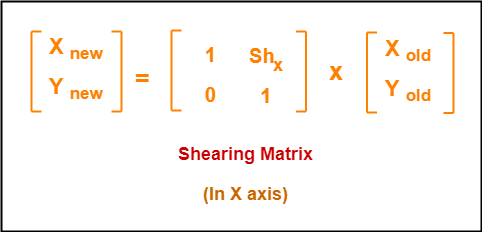For homogeneous coordinates, the above shearing matrix may be represented as a 3 x 3 matrix as-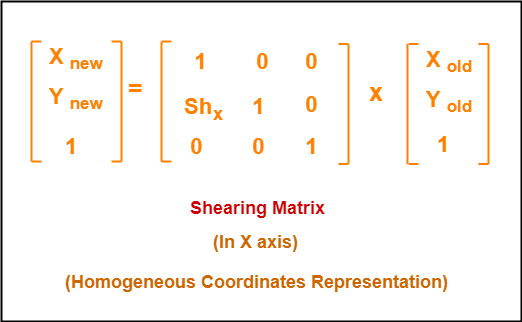### Shearing in Y Axis-

Shearing in Y axis is achieved by using the following shearing equations-

• Xnew = Xold
• Ynew = Yold + Shy x Xold

In Matrix form, the above shearing equations may be represented as-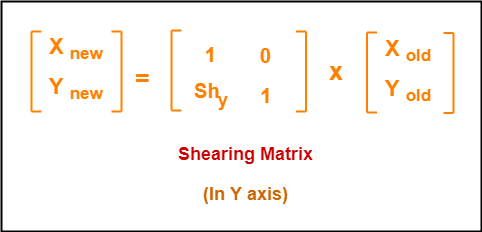For homogeneous coordinates, the above shearing matrix may be represented as a 3 x 3 matrix as-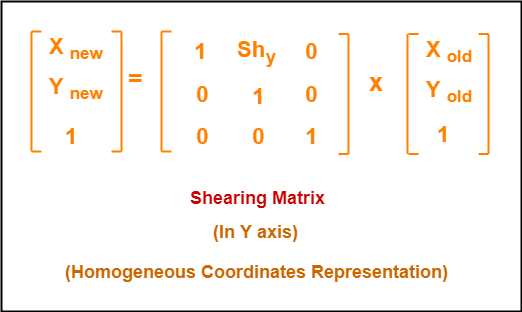## Problem-01:

Given a triangle with points (1, 1), (0, 0) and (1, 0). Apply shear parameter 2 on X axis and 2 on Y axis and find out the new coordinates of the object.

## Solution-

Given-

• Old corner coordinates of the triangle = A (1, 1), B(0, 0), C(1, 0)
• Shearing parameter towards X direction (Shx) = 2
• Shearing parameter towards Y direction (Shy) = 2

## Shearing in X Axis-

### For Coordinates A(1, 1)

Let the new coordinates of corner A after shearing = (Xnew, Ynew).

Applying the shearing equations, we have-

• Xnew = Xold + Shx x Yold = 1 + 2 x 1 = 3
• Ynew = Yold = 1

Thus, New coordinates of corner A after shearing = (3, 1).

### For Coordinates B(0, 0)

Let the new coordinates of corner B after shearing = (Xnew, Ynew).

Applying the shearing equations, we have-

• Xnew = Xold + Shx x Yold = 0 + 2 x 0 = 0
• Ynew = Yold = 0

Thus, New coordinates of corner B after shearing = (0, 0).

### For Coordinates C(1, 0)

Let the new coordinates of corner C after shearing = (Xnew, Ynew).

Applying the shearing equations, we have-

• Xnew = Xold + Shx x Yold = 1 + 2 x 0 = 1
• Ynew = Yold = 0

Thus, New coordinates of corner C after shearing = (1, 0).

Thus, New coordinates of the triangle after shearing in X axis = A (3, 1), B(0, 0), C(1, 0).

## Shearing in Y Axis-

### For Coordinates A(1, 1)

Let the new coordinates of corner A after shearing = (Xnew, Ynew).

Applying the shearing equations, we have-

• Xnew = Xold = 1
• Ynew = Yold + Shy x Xold = 1 + 2 x 1 = 3

Thus, New coordinates of corner A after shearing = (1, 3).

### For Coordinates B(0, 0)

Let the new coordinates of corner B after shearing = (Xnew, Ynew).

Applying the shearing equations, we have-

• Xnew = Xold = 0
• Ynew = Yold + Shy x Xold = 0 + 2 x 0 = 0

Thus, New coordinates of corner B after shearing = (0, 0).

### For Coordinates C(1, 0)

Let the new coordinates of corner C after shearing = (Xnew, Ynew).

Applying the shearing equations, we have-

• Xnew = Xold = 1
• Ynew = Yold + Shy x Xold = 0 + 2 x 1 = 2

Thus, New coordinates of corner C after shearing = (1, 2).

Thus, New coordinates of the triangle after shearing in Y axis = A (1, 3), B(0, 0), C(1, 2).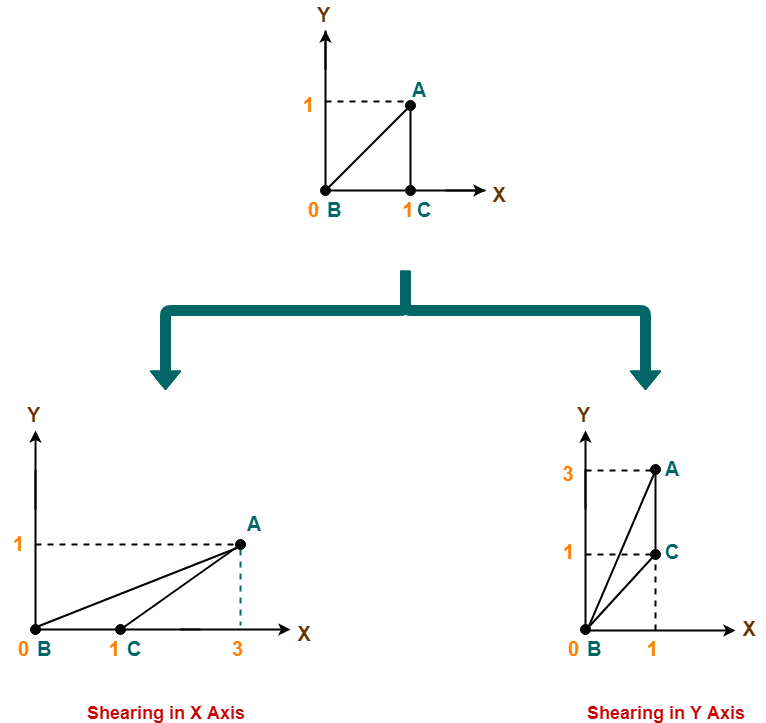To gain better understanding about 2D Shearing in Computer Graphics,

Watch this Video Lecture

Get more notes and other study material of Computer Graphics.

Watch video lectures by visiting our YouTube channel LearnVidFun.

## 2D Transformations in Computer Graphics-

We have discussed-

• Transformation is a process of modifying and re-positioning the existing graphics.
• 2D Transformations take place in a two dimensional plane.

In computer graphics, various transformation techniques are-## 2D Reflection in Computer Graphics-

• Reflection is a kind of rotation where the angle of rotation is 180 degree.
• The reflected object is always formed on the other side of mirror.
• The size of reflected object is same as the size of original object.

Consider a point object O has to be reflected in a 2D plane.

Let-

• Initial coordinates of the object O = (Xold, Yold)
• New coordinates of the reflected object O after reflection = (Xnew, Ynew)

### Reflection On X-Axis:

This reflection is achieved by using the following reflection equations-

• Xnew = Xold
• Ynew = -Yold

In Matrix form, the above reflection equations may be represented as-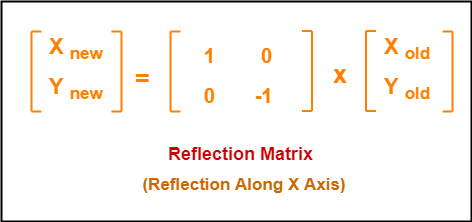For homogeneous coordinates, the above reflection matrix may be represented as a 3 x 3 matrix as-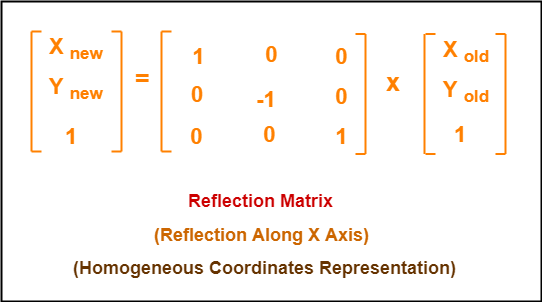### Reflection On Y-Axis:

This reflection is achieved by using the following reflection equations-

• Xnew = -Xold
• Ynew = Yold

In Matrix form, the above reflection equations may be represented as-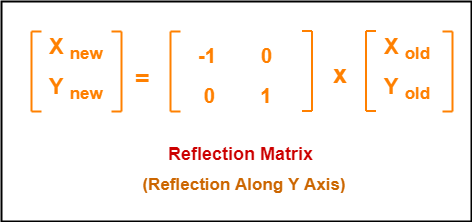For homogeneous coordinates, the above reflection matrix may be represented as a 3 x 3 matrix as-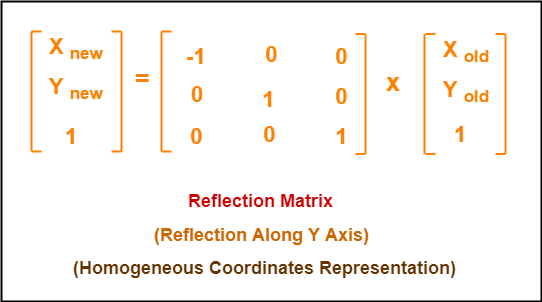## Problem-01:

Given a triangle with coordinate points A(3, 4), B(6, 4), C(5, 6). Apply the reflection on the X axis and obtain the new coordinates of the object.

## Solution-

Given-

• Old corner coordinates of the triangle = A (3, 4), B(6, 4), C(5, 6)
• Reflection has to be taken on the X axis

### For Coordinates A(3, 4)

Let the new coordinates of corner A after reflection = (Xnew, Ynew).

Applying the reflection equations, we have-

• Xnew = Xold = 3
• Ynew = -Yold = -4

Thus, New coordinates of corner A after reflection = (3, -4).

### For Coordinates B(6, 4)

Let the new coordinates of corner B after reflection = (Xnew, Ynew).

Applying the reflection equations, we have-

• Xnew = Xold = 6
• Ynew = -Yold = -4

Thus, New coordinates of corner B after reflection = (6, -4).

### For Coordinates C(5, 6)

Let the new coordinates of corner C after reflection = (Xnew, Ynew).

Applying the reflection equations, we have-

• Xnew = Xold = 5
• Ynew = -Yold = -6

Thus, New coordinates of corner C after reflection = (5, -6).

Thus, New coordinates of the triangle after reflection = A (3, -4), B(6, -4), C(5, -6).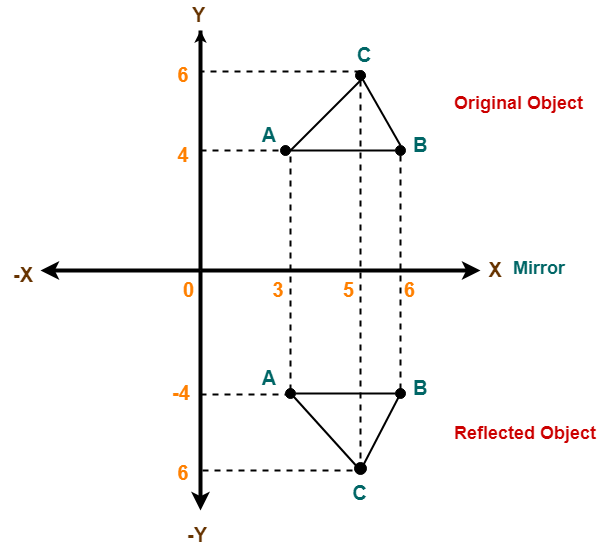## Problem-02:

Given a triangle with coordinate points A(3, 4), B(6, 4), C(5, 6). Apply the reflection on the Y axis and obtain the new coordinates of the object.

## Solution-

Given-

• Old corner coordinates of the triangle = A (3, 4), B(6, 4), C(5, 6)
• Reflection has to be taken on the Y axis

### For Coordinates A(3, 4)

Let the new coordinates of corner A after reflection = (Xnew, Ynew).

Applying the reflection equations, we have-

• Xnew = -Xold = -3
• Ynew = Yold = 4

Thus, New coordinates of corner A after reflection = (-3, 4).

### For Coordinates B(6, 4)

Let the new coordinates of corner B after reflection = (Xnew, Ynew).

Applying the reflection equations, we have-

• Xnew = -Xold = -6
• Ynew = Yold = 4

Thus, New coordinates of corner B after reflection = (-6, 4).

### For Coordinates C(5, 6)

Let the new coordinates of corner C after reflection = (Xnew, Ynew).

Applying the reflection equations, we have-

• Xnew = -Xold = -5
• Ynew = Yold = 6

Thus, New coordinates of corner C after reflection = (-5, 6).

Thus, New coordinates of the triangle after reflection = A (-3, 4), B(-6, 4), C(-5, 6).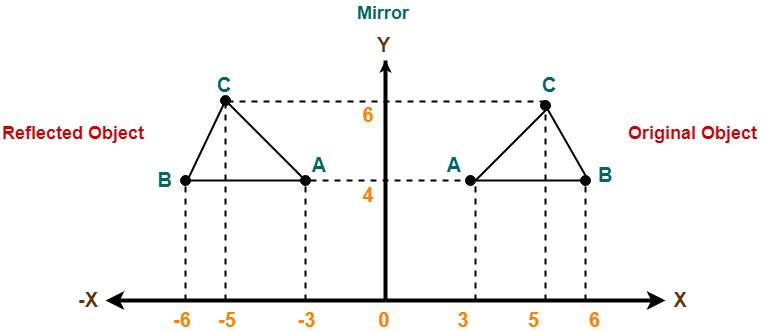To gain better understanding about 2D Reflection in Computer Graphics,

Watch this Video Lecture

Next Article- 2D Shearing in Computer Graphics

Get more notes and other study material of Computer Graphics.

Watch video lectures by visiting our YouTube channel LearnVidFun.

## 2D Transformations in Computer Graphics-

We have discussed-

• Transformation is a process of modifying and re-positioning the existing graphics.
• 2D Transformations take place in a two dimensional plane.

In computer graphics, various transformation techniques are-## 2D Scaling in Computer Graphics-

 In computer graphics, scaling is a process of modifying or altering the size of objects.

• Scaling may be used to increase or reduce the size of object.
• Scaling subjects the coordinate points of the original object to change.
• Scaling factor determines whether the object size is to be increased or reduced.
• If scaling factor > 1, then the object size is increased.
• If scaling factor < 1, then the object size is reduced.

Consider a point object O has to be scaled in a 2D plane.

Let-

• Initial coordinates of the object O = (Xold, Yold)
• Scaling factor for X-axis = Sx
• Scaling factor for Y-axis = Sy
• New coordinates of the object O after scaling = (Xnew, Ynew)

This scaling is achieved by using the following scaling equations-

• Xnew = Xold x Sx
• Ynew = Yold x Sy

In Matrix form, the above scaling equations may be represented as-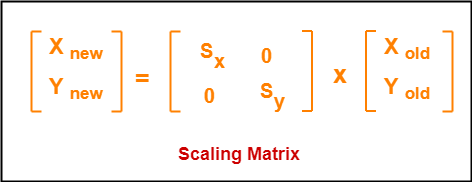For homogeneous coordinates, the above scaling matrix may be represented as a 3 x 3 matrix as-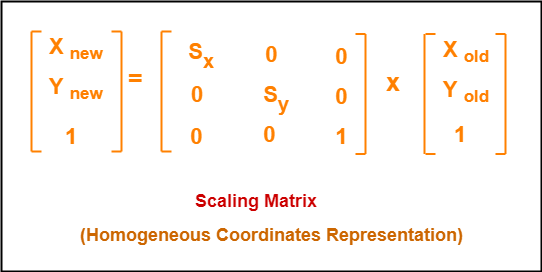## Problem-01:

Given a square object with coordinate points A(0, 3), B(3, 3), C(3, 0), D(0, 0). Apply the scaling parameter 2 towards X axis and 3 towards Y axis and obtain the new coordinates of the object.

## Solution-

Given-

• Old corner coordinates of the square = A (0, 3), B(3, 3), C(3, 0), D(0, 0)
• Scaling factor along X axis = 2
• Scaling factor along Y axis = 3

### For Coordinates A(0, 3)

Let the new coordinates of corner A after scaling = (Xnew, Ynew).

Applying the scaling equations, we have-

• Xnew = Xold x Sx = 0  x 2 = 0
• Ynew = Yold x Sy = 3 x 3 = 9

Thus, New coordinates of corner A after scaling = (0, 9).

### For Coordinates B(3, 3)

Let the new coordinates of corner B after scaling = (Xnew, Ynew).

Applying the scaling equations, we have-

• Xnew = Xold x Sx = 3  x 2 = 6
• Ynew = Yold x Sy = 3 x 3 = 9

Thus, New coordinates of corner B after scaling = (6, 9).

### For Coordinates C(3, 0)

Let the new coordinates of corner C after scaling = (Xnew, Ynew).

Applying the scaling equations, we have-

• Xnew = Xold x Sx = 3  x 2 = 6
• Ynew = Yold x Sy = 0 x 3 = 0

Thus, New coordinates of corner C after scaling = (6, 0).

### For Coordinates D(0, 0)

Let the new coordinates of corner D after scaling = (Xnew, Ynew).

Applying the scaling equations, we have-

• Xnew = Xold x Sx = 0  x 2 = 0
• Ynew = Yold x Sy = 0 x 3 = 0

Thus, New coordinates of corner D after scaling = (0, 0).

Thus, New coordinates of the square after scaling = A (0, 9), B(6, 9), C(6, 0), D(0, 0).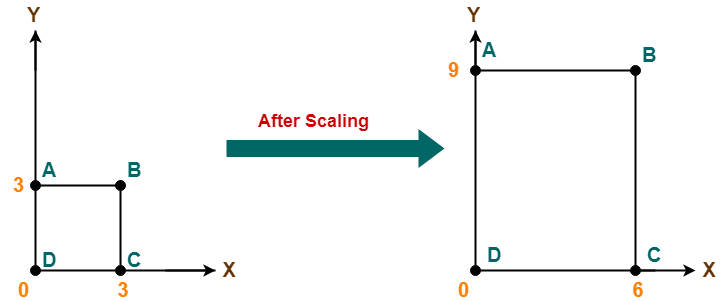To gain better understanding about 2D Scaling in Computer Graphics,

Watch this Video Lecture

Next Article- 2D Reflection in Computer Graphics

Get more notes and other study material of Computer Graphics.

Watch video lectures by visiting our YouTube channel LearnVidFun.

## 2D Transformations in Computer Graphics-

We have discussed-

• Transformation is a process of modifying and re-positioning the existing graphics.
• 2D Transformations take place in a two dimensional plane.

In computer graphics, various transformation techniques are-## 2D Rotation in Computer Graphics-

 In Computer graphics,2D Rotation is a process of rotating an object with respect to an angle in a two dimensional plane.

Consider a point object O has to be rotated from one angle to another in a 2D plane.

Let-

• Initial coordinates of the object O = (Xold, Yold)
• Initial angle of the object O with respect to origin = Φ
• Rotation angle = θ
• New coordinates of the object O after rotation = (Xnew, Ynew)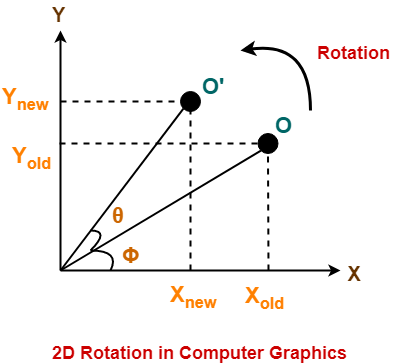This rotation is achieved by using the following rotation equations-

• Xnew = Xold x cosθ – Yold x sinθ
• Ynew = Xold x sinθ + Yold x cosθ

In Matrix form, the above rotation equations may be represented as-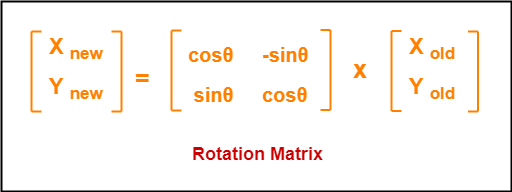For homogeneous coordinates, the above rotation matrix may be represented as a 3 x 3 matrix as-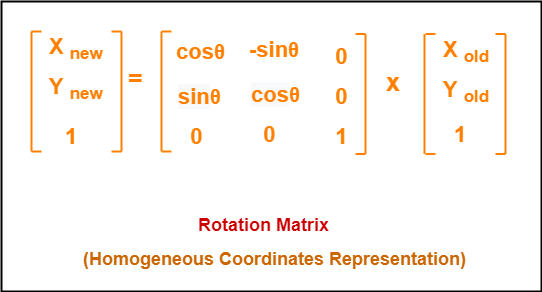## Problem-01:

Given a line segment with starting point as (0, 0) and ending point as (4, 4). Apply 30 degree rotation anticlockwise direction on the line segment and find out the new coordinates of the line.

## Solution-

We rotate a straight line by its end points with the same angle. Then, we re-draw a line between the new end points.

Given-

• Old ending coordinates of the line = (Xold, Yold) = (4, 4)
• Rotation angle = θ = 30º

Let new ending coordinates of the line after rotation = (Xnew, Ynew).

Applying the rotation equations, we have-

Xnew

= Xold x cosθ – Yold x sinθ

= 4 x cos30º – 4 x sin30º

= 4 x (√3 / 2) – 4 x (1 / 2)

= 2√3 – 2

= 2(√3 – 1)

= 2(1.73 – 1)

= 1.46

Ynew

= Xold x sinθ + Yold x cosθ

= 4 x sin30º + 4 x cos30º

= 4 x (1 / 2) + 4 x (√3 / 2)

= 2 + 2√3

= 2(1 + √3)

= 2(1 + 1.73)

= 5.46

Thus, New ending coordinates of the line after rotation = (1.46, 5.46).

Alternatively,

In matrix form, the new ending coordinates of the line after rotation may be obtained as-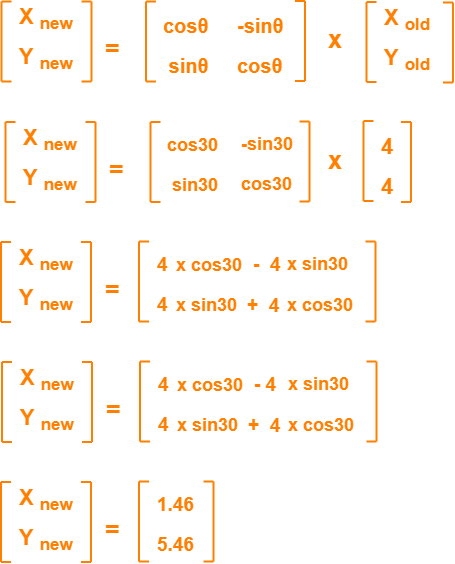Thus, New ending coordinates of the line after rotation = (1.46, 5.46).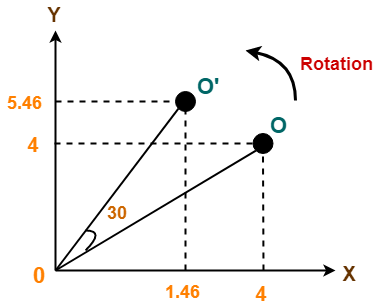## Problem-02:

Given a triangle with corner coordinates (0, 0), (1, 0) and (1, 1). Rotate the triangle by 90 degree anticlockwise direction and find out the new coordinates.

## Solution-

We rotate a polygon by rotating each vertex of it with the same rotation angle.

Given-

• Old corner coordinates of the triangle = A (0, 0), B(1, 0), C(1, 1)
• Rotation angle = θ = 90º

### For Coordinates A(0, 0)

Let the new coordinates of corner A after rotation = (Xnew, Ynew).

Applying the rotation equations, we have-

Xnew

= Xold x cosθ – Yold x sinθ

= 0 x cos90º – 0 x sin90º

= 0

Ynew

= Xold x sinθ + Yold x cosθ

= 0 x sin90º + 0 x cos90º

= 0

Thus, New coordinates of corner A after rotation = (0, 0).

### For Coordinates B(1, 0)

Let the new coordinates of corner B after rotation = (Xnew, Ynew).

Xnew

= Xold x cosθ – Yold x sinθ

= 1 x cos90º – 0 x sin90º

= 0

Ynew

= Xold x sinθ + Yold x cosθ

= 1 x sin90º + 0 x cos90º

= 1 + 0

= 1

Thus, New coordinates of corner B after rotation = (0, 1).

### For Coordinates C(1, 1)

Let the new coordinates of corner C after rotation = (Xnew, Ynew).

Xnew

= Xold x cosθ – Yold x sinθ

= 1 x cos90º – 1 x sin90º

= 0 – 1

= -1

Ynew

= Xold x sinθ + Yold x cosθ

= 1 x sin90º + 1 x cos90º

= 1 + 0

= 1

Thus, New coordinates of corner C after rotation = (-1, 1).

Thus, New coordinates of the triangle after rotation = A (0, 0), B(0, 1), C(-1, 1).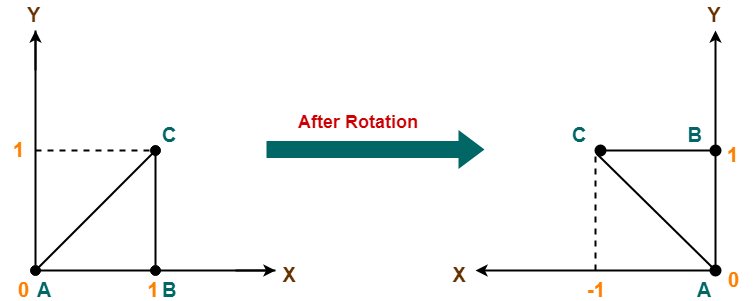To gain better understanding about 2D Rotation in Computer Graphics,

Watch this Video Lecture

Next Article- 2D Scaling in Computer Graphics

Get more notes and other study material of Computer Graphics.

Watch video lectures by visiting our YouTube channel LearnVidFun.

## 2D Transformation in Computer Graphics-

 In Computer graphics,Transformation is a process of modifying and re-positioning the existing graphics.

• 2D Transformations take place in a two dimensional plane.
• Transformations are helpful in changing the position, size, orientation, shape etc of the object.

### Transformation Techniques-

In computer graphics, various transformation techniques are-## 2D Translation in Computer Graphics-

 In Computer graphics,2D Translation is a process of moving an object from one position to another in a two dimensional plane.

Consider a point object O has to be moved from one position to another in a 2D plane.

Let-

• Initial coordinates of the object O = (Xold, Yold)
• New coordinates of the object O after translation = (Xnew, Ynew)
• Translation vector or Shift vector = (Tx, Ty)

Given a Translation vector (Tx, Ty)-

• Tx defines the distance the Xold coordinate has to be moved.
• Ty defines the distance the Yold coordinate has to be moved.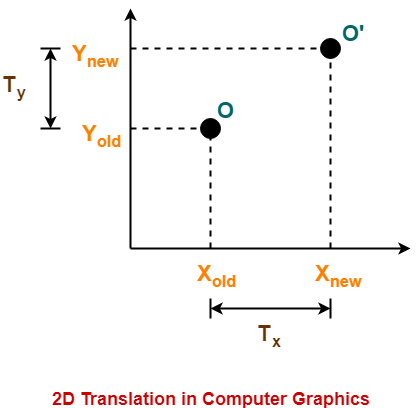This translation is achieved by adding the translation coordinates to the old coordinates of the object as-

• Xnew = Xold + Tx     (This denotes translation towards X axis)
• Ynew = Yold + Ty     (This denotes translation towards Y axis)

In Matrix form, the above translation equations may be represented as-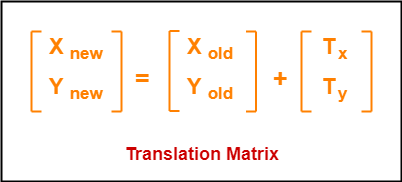• The homogeneous coordinates representation of (X, Y) is (X, Y, 1).
• Through this representation, all the transformations can be performed using matrix / vector multiplications.

The above translation matrix may be represented as a 3 x 3 matrix as-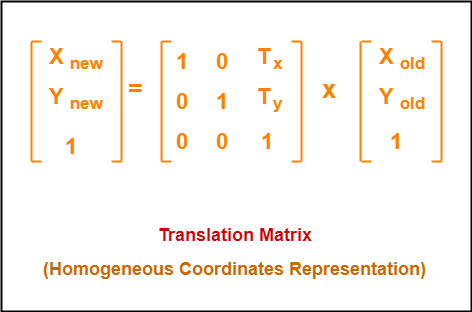## Problem-01:

Given a circle C with radius 10 and center coordinates (1, 4). Apply the translation with distance 5 towards X axis and 1 towards Y axis. Obtain the new coordinates of C without changing its radius.

## Solution-

Given-

• Old center coordinates of C = (Xold, Yold) = (1, 4)
• Translation vector = (Tx, Ty) = (5, 1)

Let the new center coordinates of C = (Xnew, Ynew).

Applying the translation equations, we have-

• Xnew = Xold + Tx = 1 + 5 = 6
• Ynew = Yold + Ty = 4 + 1 = 5

Thus, New center coordinates of C = (6, 5).

Alternatively,

In matrix form, the new center coordinates of C after translation may be obtained as-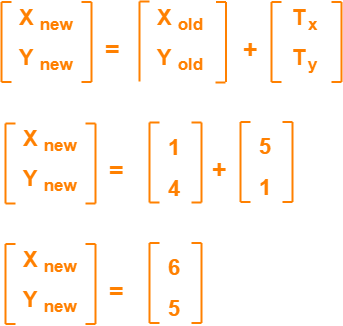Thus, New center coordinates of C = (6, 5).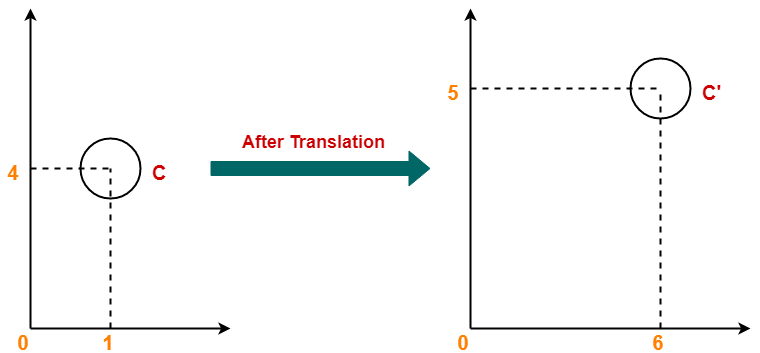## Problem-02:

Given a square with coordinate points A(0, 3), B(3, 3), C(3, 0), D(0, 0). Apply the translation with distance 1 towards X axis and 1 towards Y axis. Obtain the new coordinates of the square.

## Solution-

Given-

• Old coordinates of the square = A (0, 3), B(3, 3), C(3, 0), D(0, 0)
• Translation vector = (Tx, Ty) = (1, 1)

### For Coordinates A(0, 3)

Let the new coordinates of corner A = (Xnew, Ynew).

Applying the translation equations, we have-

• Xnew = Xold + Tx = 0 + 1 = 1
• Ynew = Yold + Ty = 3 + 1 = 4

Thus, New coordinates of corner A = (1, 4).

### For Coordinates B(3, 3)

Let the new coordinates of corner B = (Xnew, Ynew).

Applying the translation equations, we have-

• Xnew = Xold + Tx = 3 + 1 = 4
• Ynew = Yold + Ty = 3 + 1 = 4

Thus, New coordinates of corner B = (4, 4).

### For Coordinates C(3, 0)

Let the new coordinates of corner C = (Xnew, Ynew).

Applying the translation equations, we have-

• Xnew = Xold + Tx = 3 + 1 = 4
• Ynew = Yold + Ty = 0 + 1 = 1

Thus, New coordinates of corner C = (4, 1).

### For Coordinates D(0, 0)

Let the new coordinates of corner D = (Xnew, Ynew).

Applying the translation equations, we have-

• Xnew = Xold + Tx = 0 + 1 = 1
• Ynew = Yold + Ty = 0 + 1 = 1

Thus, New coordinates of corner D = (1, 1).

Thus, New coordinates of the square = A (1, 4), B(4, 4), C(4, 1), D(1, 1).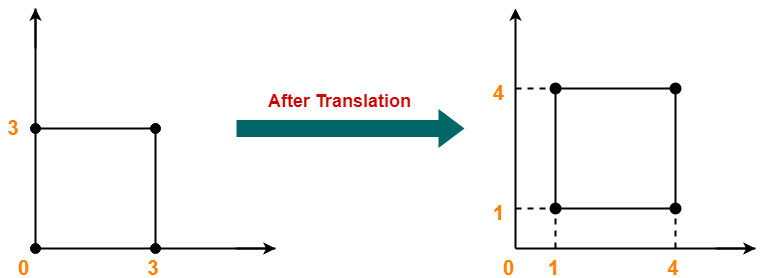To gain better understanding about 2D Translation in Computer Graphics,

Watch this Video Lecture

Next Article- 2D Rotation in Computer Graphics

Get more notes and other study material of Computer Graphics.

Watch video lectures by visiting our YouTube channel LearnVidFun.